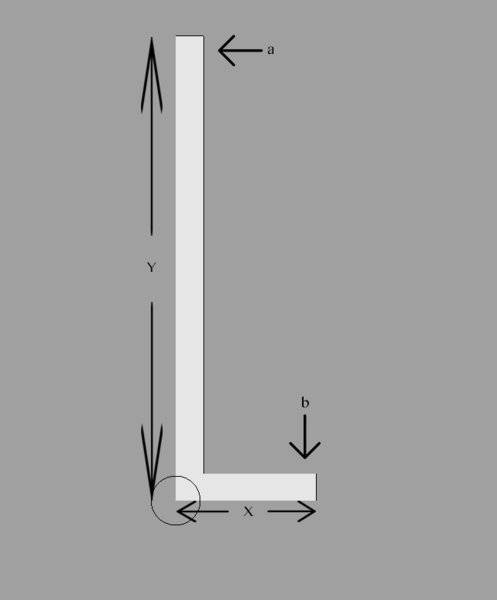# Counterweight calculation

• Alistair Vowles
In summary, the bar will need more counterweight to not pivot if the force being applied at point 'a' is wind resistance.

#### Alistair Vowles

I hope this will be an easy one to answer that I could just use a little help with.

I have an L shaped bar where 'X' is on a flat surface. The pivot point is the circle.

Can someone help me with a formula to calculate the force needed at point 'b' , given a certain force applied at point 'a' , to not allow the bar to pivot.

Force 'a' will be a varying force (wind) so I want to make sure I have enough counterweight for force 'b' to allow for this variable.

The fig is not to scale and I plan to have the length of 'X' to be adjustable to decrease the force needed at point 'b' .

I hope this makes sense and thank you for the help !#### Attachments

b=a*y/x

If you want an explanation...

If you don't want it to move/accelerate then the net torque must be zero.

Let's define counter clockwise as positive. Then...

ay - bx = 0

Rearrange to give...

ay = bx
Then
b = ay/x

Thankyou very much. This is perfect and exactly what I needed.

Um, force being wind resistance is spread all the way along y but can be taken as applying at the average point (y/2) (roughly) to simplify the calculation.

So, b = ay/x/2

Be aware though that if the base is on the ground or a surface, there is a velocity gradient increasing with distance from the surface which is the reason for (roughly). If the structure is very tall, this could be a significant consideration. If it's an engineering question, you will need at least double, probably quadruple (according to regulation) b to provide a safety factor. This is especially important for aero forces as they are proportional to velocity squared and wind is gusty.

You may already have taken all this into account, it's not stated.

## 1. What is a counterweight calculation?

A counterweight calculation is a process used to determine the amount of weight needed to balance or stabilize an object or system. It involves considering the weight, dimensions, and center of gravity of the object, as well as external factors such as force and motion.

## 2. Why is counterweight calculation important?

Counterweight calculation is important because it ensures the stability and safety of an object or system. Without proper counterweight calculations, an object may be at risk of tipping over or becoming unbalanced, which can lead to accidents and damage.

## 3. What factors are considered in a counterweight calculation?

The weight, dimensions, and center of gravity of the object are primary factors considered in a counterweight calculation. Other factors may include external forces, motion, and the environment in which the object will be used.

## 4. What types of objects require counterweight calculations?

Counterweight calculations are commonly used in construction, engineering, and manufacturing industries for large structures such as cranes, bridges, and buildings. They may also be used in smaller-scale applications, such as balancing a bicycle or a door.

## 5. How is a counterweight calculation performed?

A counterweight calculation is typically performed using mathematical equations and formulas that take into account the weight and dimensions of the object, its center of gravity, and external factors. Advanced software programs may also be used to assist with the calculation process.